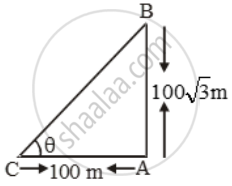# A tower is 100√3 metres high. Find the angle of elevation of its top from a point 100 metres away from its foot. - Mathematics

Sum

A tower is 100√3 metres high. Find the angle of elevation of its top from a point 100 metres away from its foot.

#### Solution

Let AB be the tower of height 100√3 metres, and let C be a point at a distance of 100 metres from the foot of the tower.Let θ be the angle of elevation of the top of the tower from point C.

In ∆CAB, we have

\tan \theta =\frac{AB}{AC}

\Rightarrow \tan \theta =\frac{100\sqrt{3}}{100}=\sqrt{3}

⇒ θ = 60º

Hence, the angle of elevation of the top of the tower from a point 100 metres away from its foot is 60º.

Concept: Heights and Distances
Is there an error in this question or solution?